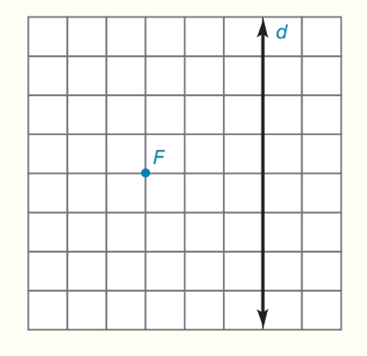Chapter 7.CT, Problem 17CT### Elementary Geometry for College St...

6th Edition
Daniel C. Alexander + 1 other
ISBN: 9781285195698

#### Solutions

Chapter
Section### Elementary Geometry for College St...

6th Edition
Daniel C. Alexander + 1 other
ISBN: 9781285195698
Textbook Problem
1 views

# Sketch the parabola that has directrix d and focus F.To determine

To sketch:

The parabola that has directrix d and focus F.

Explanation

Calculation:

A parabola is the locus of points that are equidistant t from a fixed line and a fixed point not on that line.

Given:

Draw FAd at point A. Note that FA is the axis of symmetry of the parabola. Locate the midpoint of FA, which is also the vertex V of the parabola.

Through F, draw a line parallel to d. With a compass, measure the distance FA and mark off arcs of that length on the parallel line (on both sides of F)

### Still sussing out bartleby?

Check out a sample textbook solution.

See a sample solution

#### The Solution to Your Study Problems

Bartleby provides explanations to thousands of textbook problems written by our experts, many with advanced degrees!

Get Started

#### Solve the equations in Exercises 126. 2(x21)x2+1x4x2+1x2+1=0

Finite Mathematics and Applied Calculus (MindTap Course List)

#### Find the limit. limt2t24t38

Single Variable Calculus: Early Transcendentals

#### Solve for x: ln(e + x) = 1. a) 0 b) 1 c) e d) ee e

Study Guide for Stewart's Single Variable Calculus: Early Transcendentals, 8th